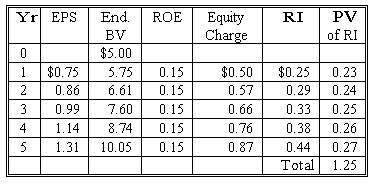### Why should I choose AnalystNotes?

AnalystNotes specializes in helping candidates pass. Period.

##### Subject 6. Multistage Residual Income Valuation
A multistage approach can be used when residual income is forecast for a certain time horizon and a terminal value based on continuing residual income is estimated at the end of the time horizon. Continuing residual income is the value of residual income after the time horizon (initial time frame).

The value of continuing residual income depends on the assumptions regarding its pattern. Frequently, one of the following assumptions is made:

• RI continues indefinitely > 0.
Economic profits are expected to persist even after the forecast horizon, although at some lower level.

• RI = 0 from terminal year onward.
Within the forecast horizon, other companies identify opportunities for economic earnings and enter the industry, driving the ROE down to the cost of equity by the terminal period.

• RI declines to zero as ROE drops to the cost of equity.
After the forecast horizon, other companies identify opportunities for economic earnings and enter the industry, driving the ROE down to the cost of equity at some point in the future. Some persistence factor may be assumed to account for the fading of continuing residual income after the forecast horizon.

• RI reflects a decline in ROE to a long-run average level.
Significant barriers exist for entry of other companies in the industry even in the long- term. If a company continues to have some kind of monopoly indefinitely, ROE will gradually converge to some constant level. It then becomes a perpetuity.

Model of ROE Converging to r

• 0< persistence factor(ω) < 1.
• Low ω => continuing RI falls quickly => lower valuation.
• High ω => continuing RI falls slowly => higher valuation.

Factors suggesting higher ω:

• Low dividend payout.
• High RI industry persistence.

Factors influencing lower ω:

• High ROE.
• Large special items.
• Large accounting accruals.

Example

• Expected ROE over 5 years = 15% for the next 5 years.
• Beginning BV = \$5.00 per share.
• Required rate of return = 10%.
• The firm pays no dividends.
• Continuing RI = 0 after 5 years.
• Assume all earnings are reinvested.V0 = B0 + sum of discounted RIs + PV of continuing RI (PV of terminal value) = \$5.00 + \$1.25 + \$0 = \$6.25.

Suppose instead RI remains constant after year 5 at \$0.44.
V0 = \$5.00 + \$1.25 + (\$0.44/0.1)/1.15 = \$8.98.

Suppose instead continuing RI persistence factor is 0.40.
EPS6 = \$10.05 x 0.15 = \$1.51.
Equity charge = \$10.05 x 0.1 = \$1.00.
RI6 = \$1.51 - \$1.00 = \$0.51.
V0 = \$5.00 + \$1.25 + [\$0.51 / (1 + 0.1 - 0.4)] / 1.15 = \$6.70.

User Comment
TheCFAGuy you don't need the table for the first equation. because all of the return is reinvested it's just compound growth, discounted back from year 5 to today:

V0 = [ BV0 * (1 + ROE)^t ] / [ (1+r)^t ]
V0 = [5*(1.15)^5] / [(1.1)^5]
V0 = 6.244

and if all earnings aren't reinvested (i.e. retention ration<1) then change out ROE for ROE after payout (where PO = payout ratio):

ROE after payout = 1 + ROE - (ROE*PO)

for the persistence factor, where EPS6 = BV5*ROE, just make BV5 = BV0*(1+ROE)^5 and your gucci. so really no tables needed at all. even with a payout ratio you just adjust the ROE that you will use to calculate future BV (but not the ROE you use to calculate EPS).
davidt876 *ROE after payout = ROE - (ROE*PO)
davidt876 and actually ROE after payout = ROE*b, where b = retention ratio